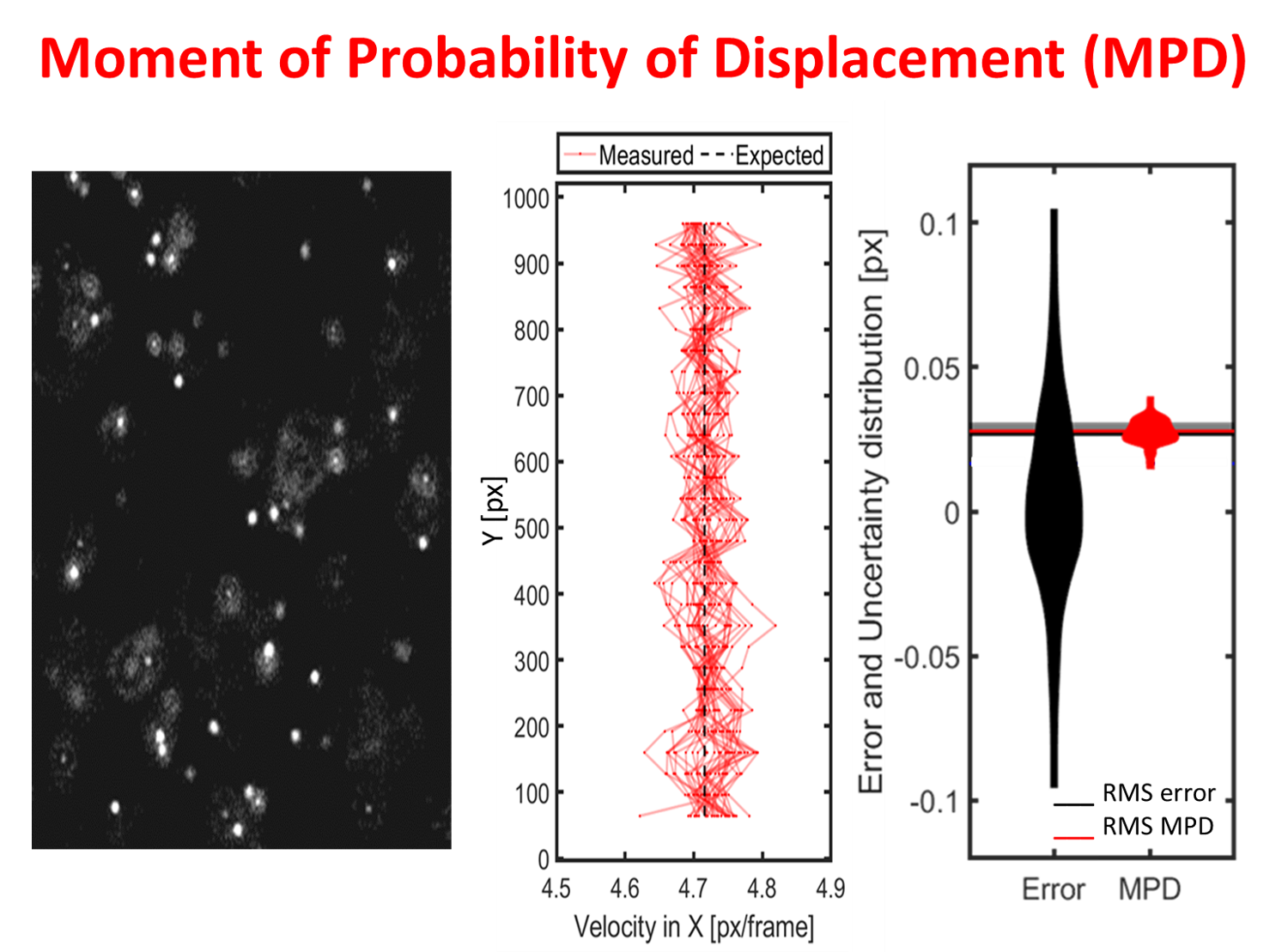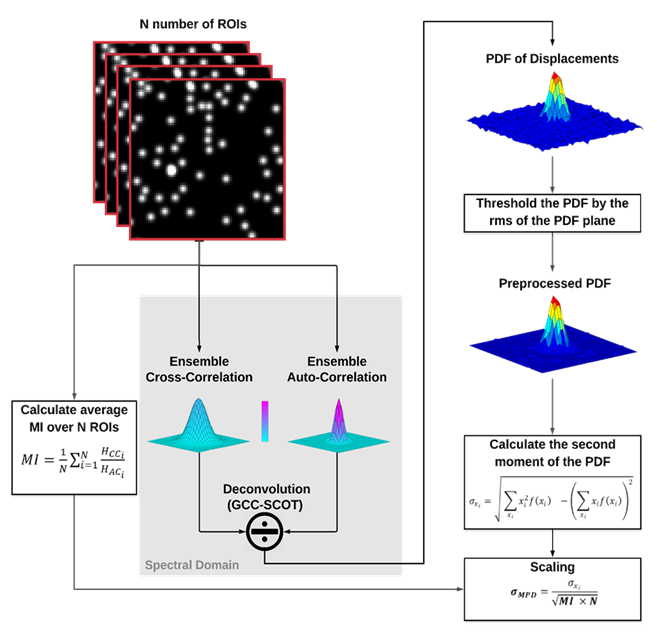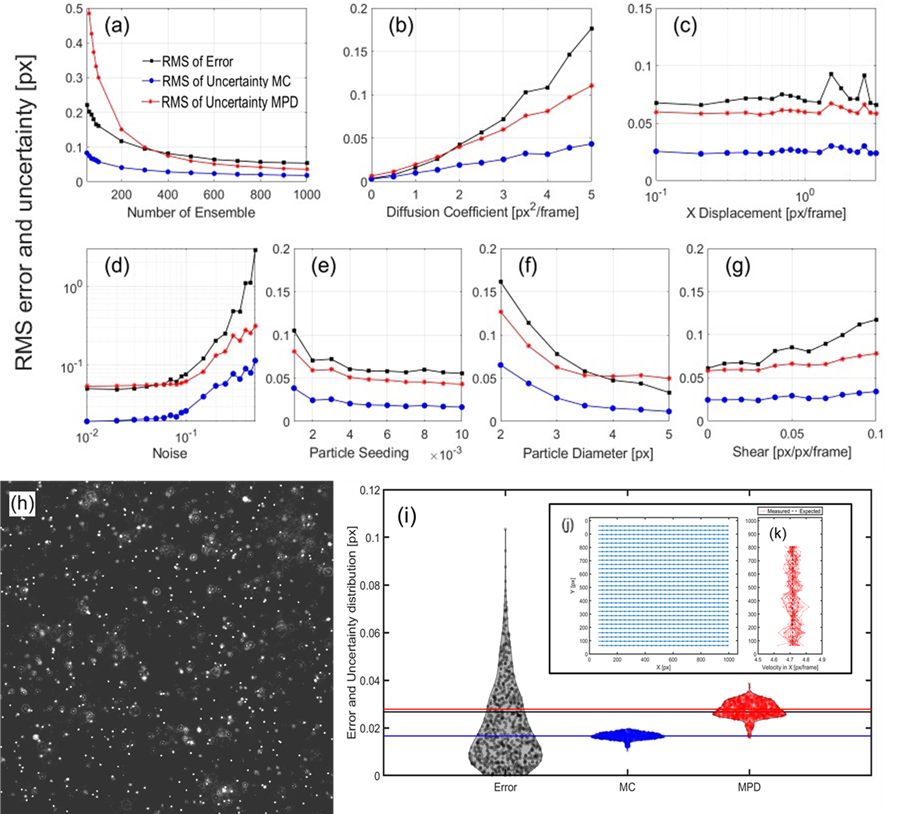Uncertainty estimation for ensemble particle image velocimetry
17434## 28 May Uncertainty estimation for ensemble particle image velocimetry

Particle Image Velocimetry (PIV) is a widely used non-invasive approach to finding a fluid flow velocity using tracer particles. Performing PIV measurement where the Signal-to-Noise Ratio (SNR) in the correlation plane is low can be unreliable and challenging. To address this issue, ensemble PIV has been developed that improves the SNR in the correlation plane by calculating the ensemble average of many PIV correlations in an interrogation region and therefore achieves a reliable velocity measurement [6–9].

Despite the utility of ensemble PIV, uncertainty quantification for this type of measurement has not been studied. The existing uncertainty quantification algorithms for PIV are developed and used only for instantaneous PIV measurement and do not account for the improved SNR in ensemble PIV. So, a generalized and reliable method for uncertainty quantification for ensemble PIV is needed.

We introduce a novel method for estimating the uncertainty directly from the probability density function of displacements found by deconvolving the ensemble cross-correlation from the ensemble autocorrelation. We then find the second moment of the probability density function and apply a scaling factor to report the uncertainty in the velocity measurement. We call this method the moment of the probability of displacement (MPD). With synthetic and experimental images, we show that predicted uncertainties with MPD agree well with the expected root mean square (RMS) of the error in the velocity measurements over a wide range of image and flow conditions.Figure 1.  MPD algorithm for estimating uncertainty in ensemble PIV. MPD estimates the uncertainty directly from the probability density function of displacements found by deconvolving the ensemble cross-correlation from the ensemble autocorrelation. It then finds the second moment of the probability density function and applies a scaling factor to report the uncertainty in the velocity measurement.Figure 2 RMS error and uncertainty from MPD and one of the existing instantaneous uncertainty methods the Moment of Correlation (MC) for different elemental error sources through synthetic images: (a) the number of ensemble correlations, (b) diffusion coefficient, i.e. randomness, (c) particle displacement in the X direction, (d) the noise percentage, (e) particle seeding density, (f) particle image diameter, (g) shear rate, (h)Experimental demonstration of MPD performance with micro-PIV data. (i) Error and uncertainty distribution for the µPIV experiment. Black corresponds to the error distribution error and blue and red show the MC and MPD, respectively. Horizontal lines show the RMS of the corresponding distribution with the same color and the horizontal gray shadow shows the uncertainty of the RMS error. (j) Measured vector field. (k) Expected vs. measured velocity in the flow direction.